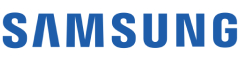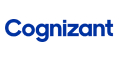New update is available. Click here to update.
Topics

Moderate0/80
Average time to solve is 30m+16 more companies

## Problem statement

For a given Singly Linked List of integers, sort the list using the 'Merge Sort' algorithm.

Detailed explanation ( Input/output format, Notes, Images )
Constraints :
``````1 <= N <= 10^5
1 <= DATA <= 10^9

Where 'DATA' denotes the value of node of Linked List.

Time Limit: 1sec
``````
Sample Input 1 :
``````1 4 5 2 -1
``````
Sample Output 1 :
``````1 2 4 5
``````
Sample Input 2 :
``````10 9 8 7 6 5 4 -1
``````
Sample Output 2 :
``````4 5 6 7 8 9 10
``````Console